Geometry

# Circle Properties: Level 1 Challenges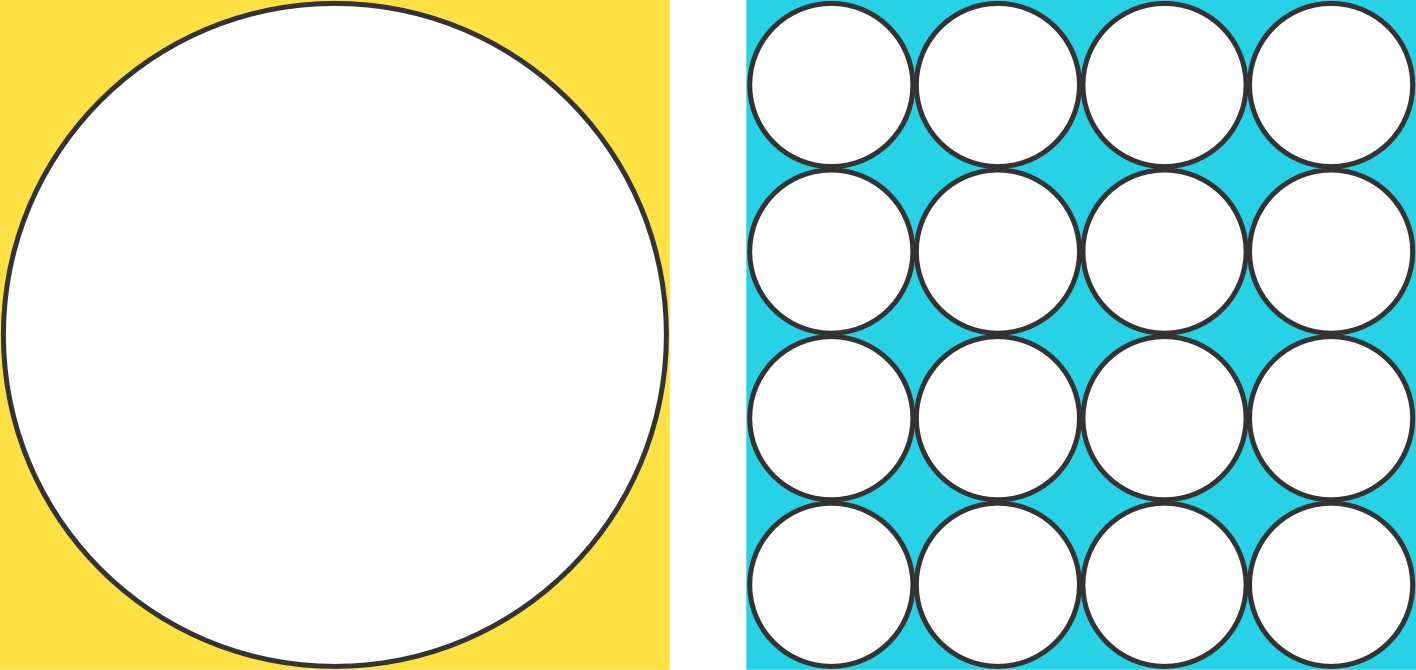In the above figure, which shaded area is larger?

The small circles all have the same size.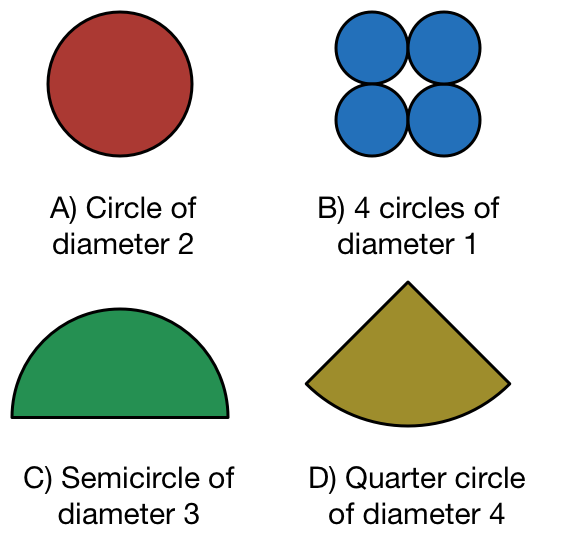Which of these shapes has an area that is different from the rest?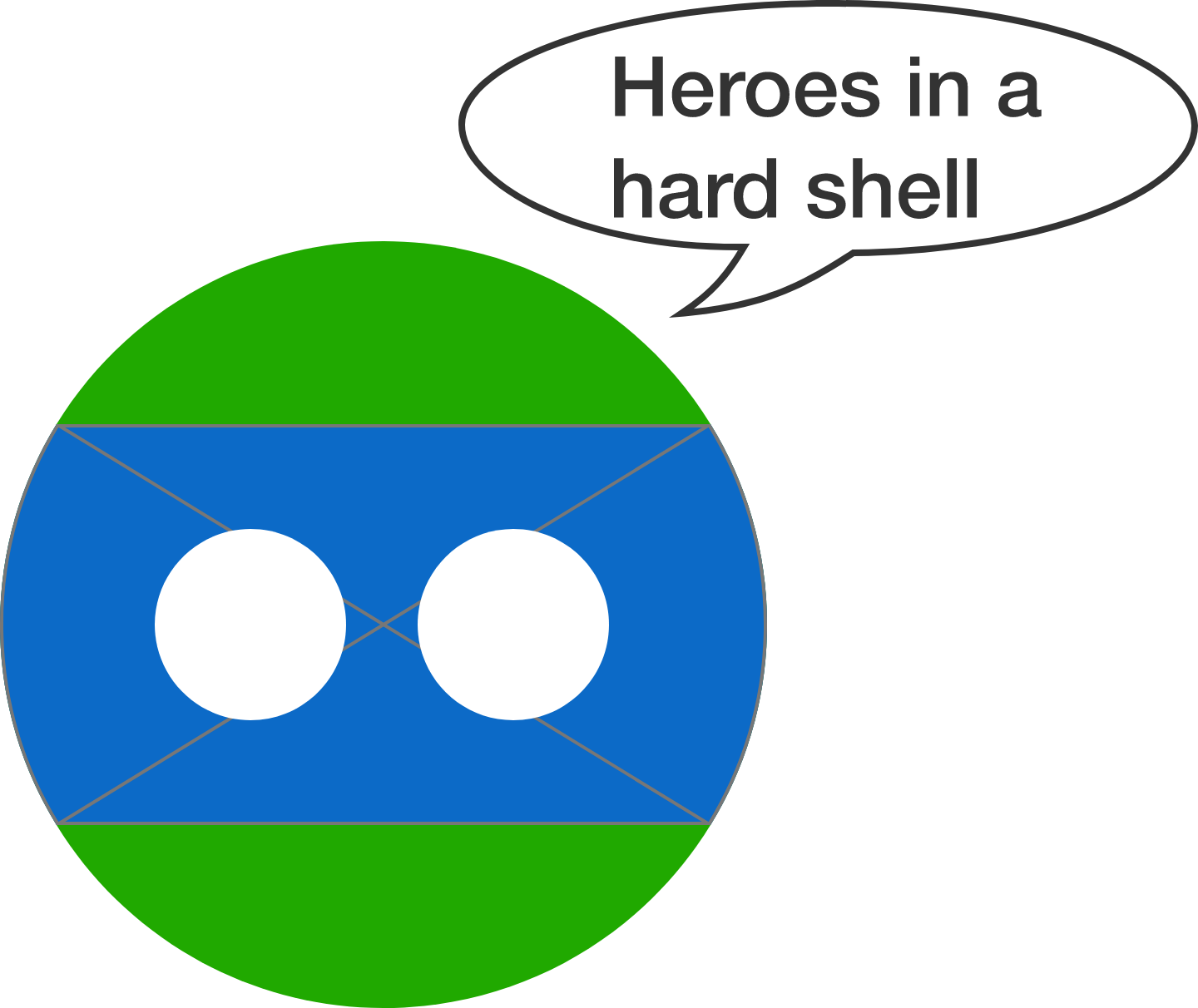When sketching out Leonardo Da Turtle, I started out with a green circle of radius 2, and then added a blue band across his eyes. The circular arcs of the blue band each subtend an angle of 60 degress at the center. And 2 triangles to fill in the gap. Finally, I added eyes of diameter 1.

What is the (visible) area of the blue band?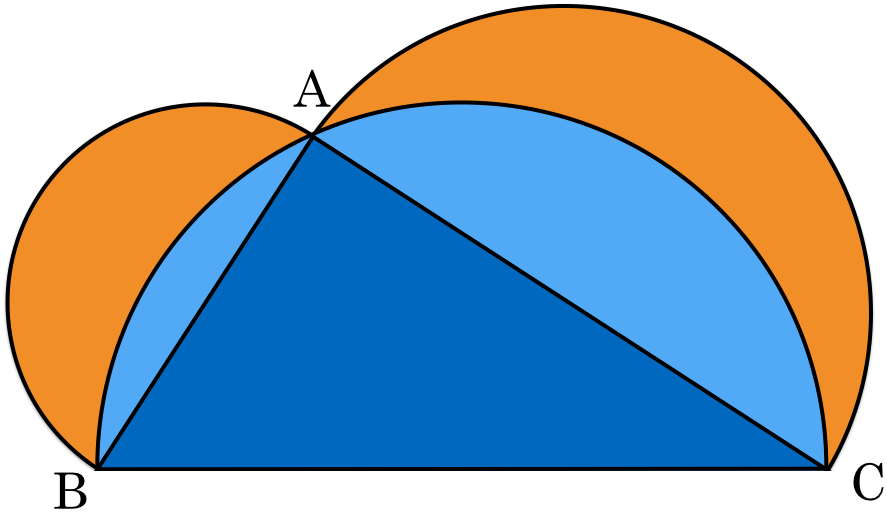Consider a triangle $ABC$ with right angle $A$, $BC=5$ and $AC=4.$ Three semicircles are drawn as in the figure above. Find the sum of the areas of the orange shaded regions.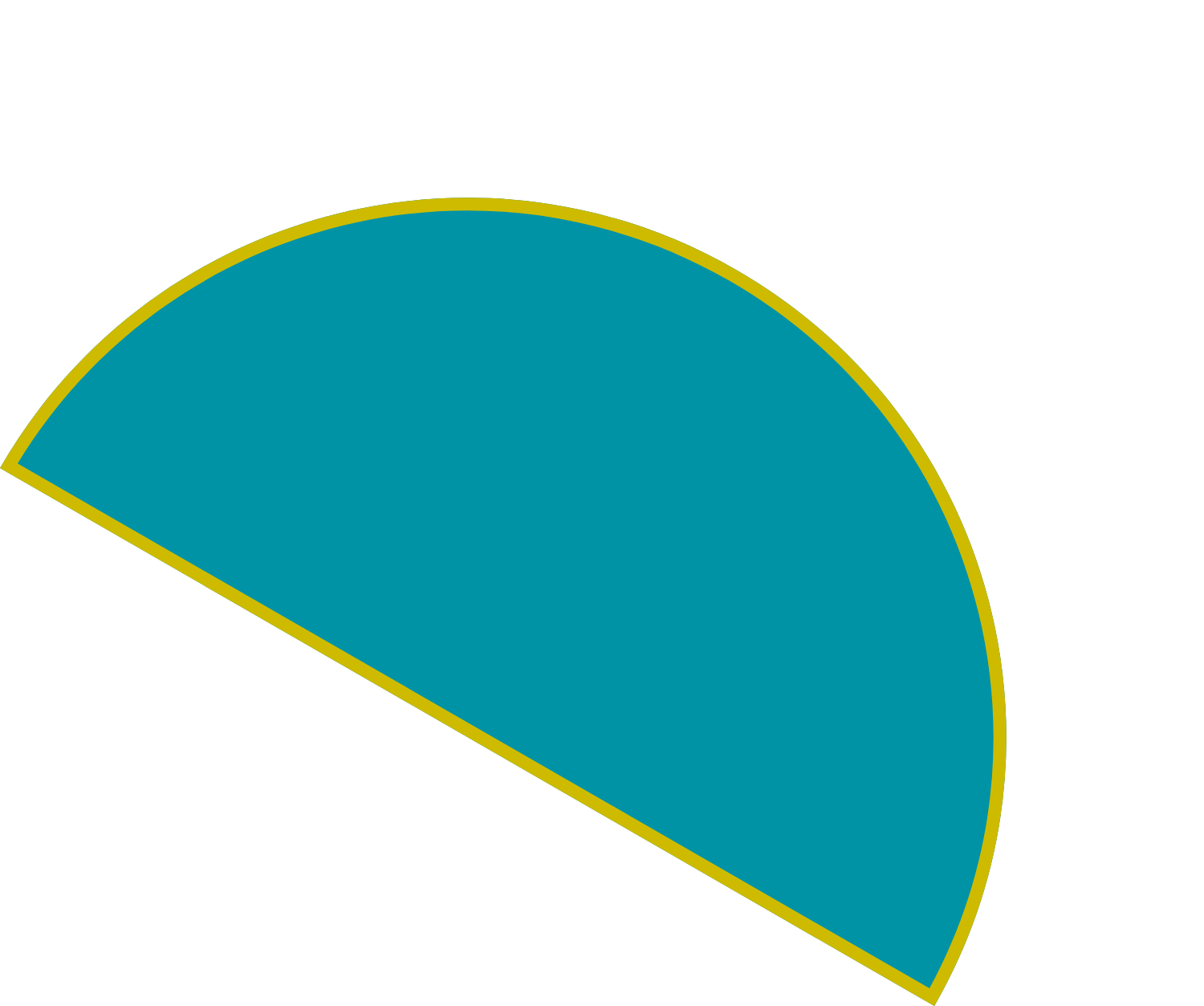What is the perimeter of a semicircle with radius 7?

Use the approximation $\pi \approx 22/7$.

×